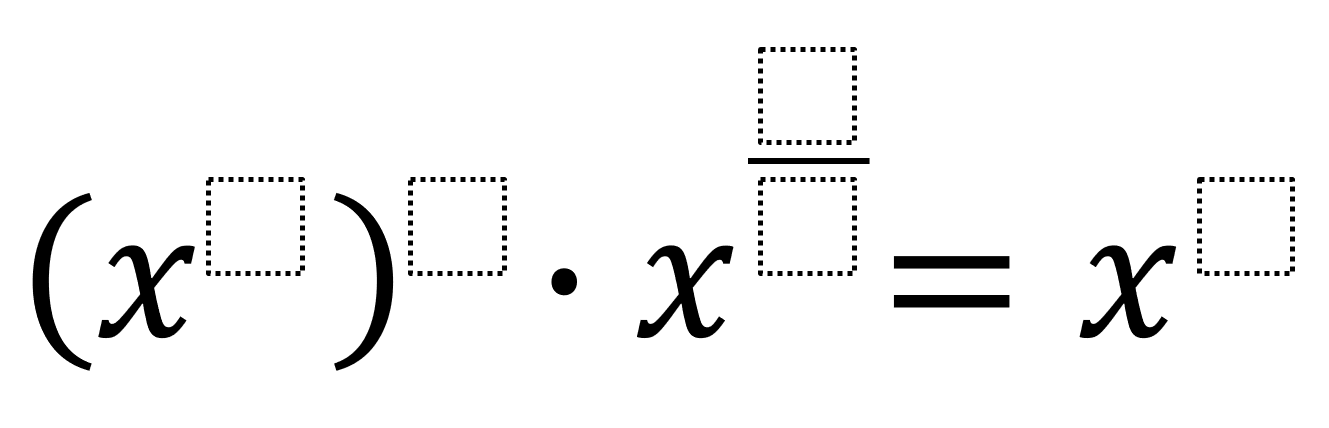# Properties of Exponents 4

Directions: Using the integers -9 to 9 at most one time each, fill in the boxes to make an equation where the product’s exponent has the greatest possible value.### Hint

How do you know when to multiply, divide, subtract, or add the exponents’ value?

There are many possible answers that make the product’s exponent equal to 9 including:
(x^3)^5 · x^(-6/1) = x^9

Thanks to everyone in the comments who pointed out that my original answer was wrong.

Source: Robert Kaplinsky

## Imaginary Solutions to a Quadratic Equation

Directions: Using the digits 1 to 9 at most one time each, place a digit …

1.x^15 . x^(1/6) is not equal to x^9 you have to add the exponent which leaves you with an exponent of 15 1/6

•I agree Heidi. If the original problem said using from -9 to 9, then a person can use -6/1 rather than 1/6 (which are not the same). This would result in an answer of x^9, assuming everything else remained the same respectively.

•If you keep it from the digits 1 to 9 a maximum of once each, you could say (x^5)^1 * x^(8/2) = x^9 or (x^1)^5 * x^(8/2) = x^9. There are some other possibilities that will result in x^9. I can think of 4 more that will also result in x^9 with the original restrictions, so a great way to increase the rigor, develop conceptual understanding, and assess numeracy skills would be to identify as many solutions as possible that result in a product with the greatest exponent.

•LOL, I got my own problem wrong for the same misconception I was trying to find out if kids had. Thanks everyone. I will fix it now.

2.The answer key is definitely wrong!

My tutoree student found a solution to end up with 9 though!

(x^1)^5 x^(8/2)= x^9

•LOL, I got my own problem wrong for the same misconception I was trying to find out if kids had. Thanks everyone. I will fix it now.